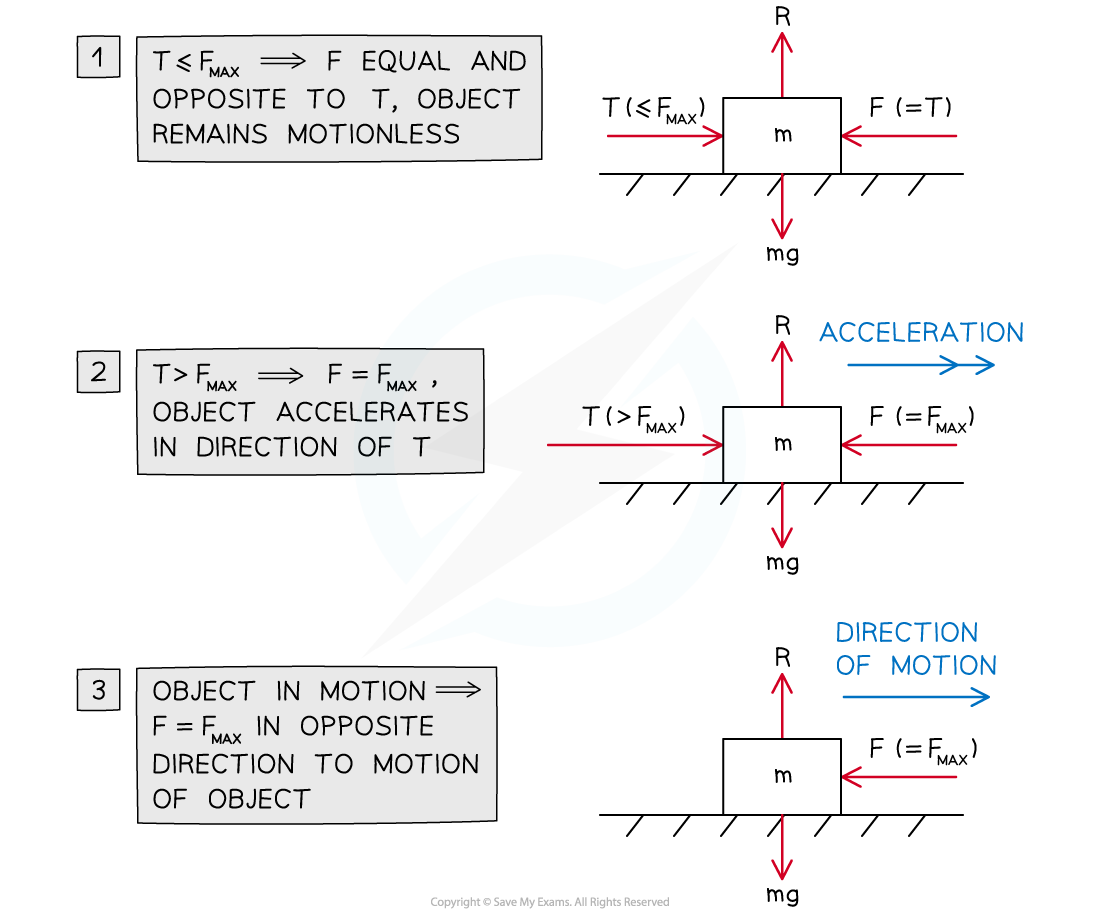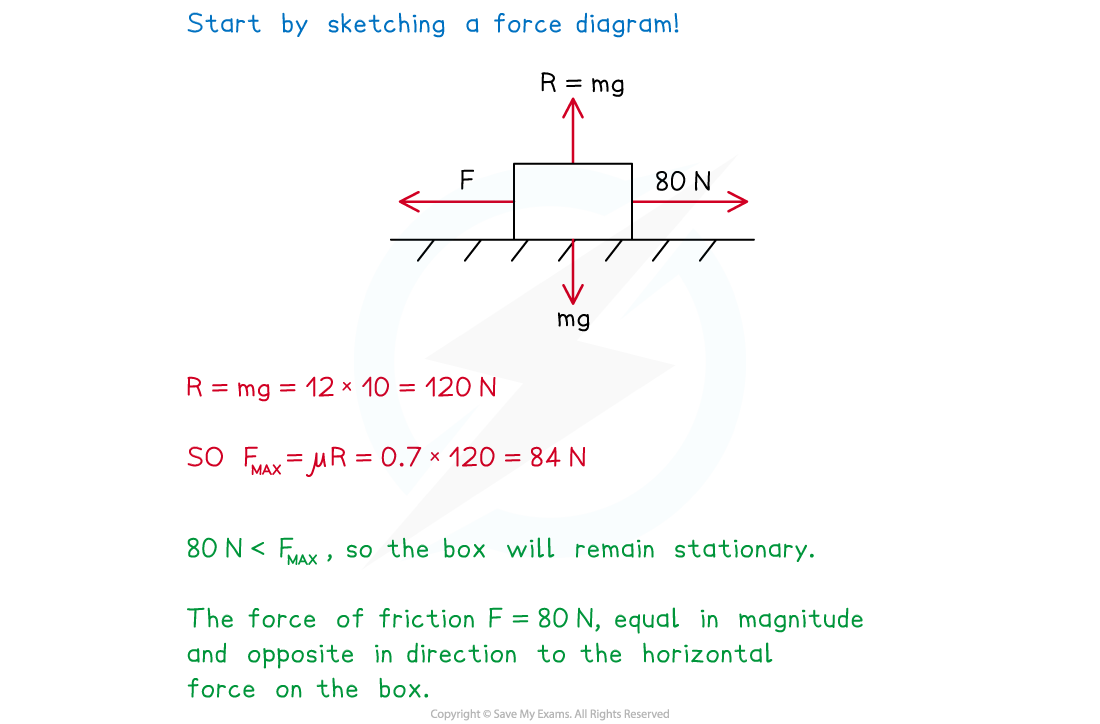# CIE A Level Maths: Mechanics复习笔记3.3.2 Coefficient of Friction

### Coefficient of Friction

#### What is friction?

• Friction is a force between a surface and an object that acts to resist motion of the object across the surface
• On a horizontal surface with no forces acting on a stationary object other than gravity and the surface's normal reaction force, there is no force of friction
• If an additional force acts on the object parallel to the surface then friction manifests as a force in the opposite direction
• Friction always acts in a direction parallel to the surface
• Friction acts in the opposite direction to the resultant force that would cause the object to move parallel to the surface
• Up to a certain limiting value (see next section) the force of friction prevents motion entirely
• Beyond that limiting value friction is a force of constant magnitude in the opposite direction to the motion of the object

#### What Is the coefficient of friction?

• The magnitude of the frictional force satisfies the inequality:

F ≤ μR

where F is the force of friction, R is the normal reaction force between the object and the surface, and μ is the coefficient of friction between the object and the surface

• If there is no friction then μ= 0 and the surface is smooth
• Otherwise μ > 0
• The maximum possible frictional force is given by the equation

FMAX = μR

where FMAX is the maximum possible force of friction, and R and μ are as above

• FMAX serves as a limiting value for mechanics problems involving friction
1. If the object is stationary and the resultant of other parallel forces to the surface is less than or equal to FMAX then
• the object remains stationary
• the frictional force is always equal and opposite to the resultant of the other horizontal forces
• if the resultant of other horizontal forces is equal to FMAX then the object is said to be in limiting equilibrium
2. If the object is stationary and the resultant of other horizontal forces becomes greater than FMAX then
• the object begins to accelerate in the direction of the resultant force
• the frictional force is equal to FMAX with a direction always opposite to that of the object's motion
3. If the object is in motion parallel to the surface then (regardless of any other forces) the frictional force will always be equal to FMAX with a direction opposite to that of the object's motion#### Worked Example

A box with a mass of 12 kg is at rest on a horizontal floor.  The coefficient of friction between the box and the floor is 0.7 .  A horizontal force of 80 N is applied to the box.  Describe the force of friction in this situation and determine whether or not the box will begin to move.#### Exam Tip

• Always draw a force diagram and label it clearly.
• Look out for the words smooth and rough in mechanics problems involving an object moving (or potentially moving) along a surface:
• If the surface is described as smooth then you can ignore friction in the problem (i.e μ= 0)
• If the surface is described as rough than you need to include the force of friction in solving the problem.
• If a friction question states that an object is on the point of moving that means that the object is in limiting equilibrium.